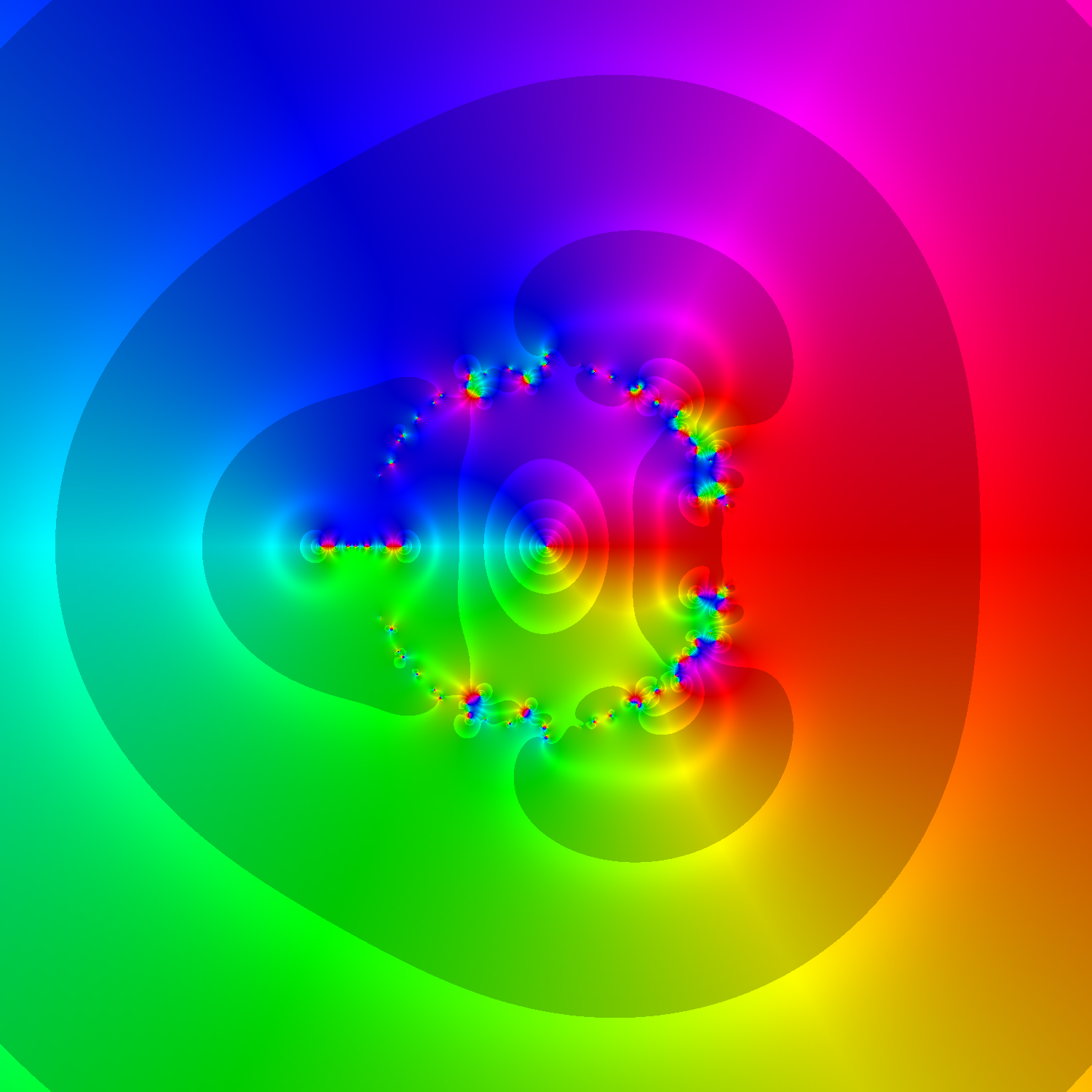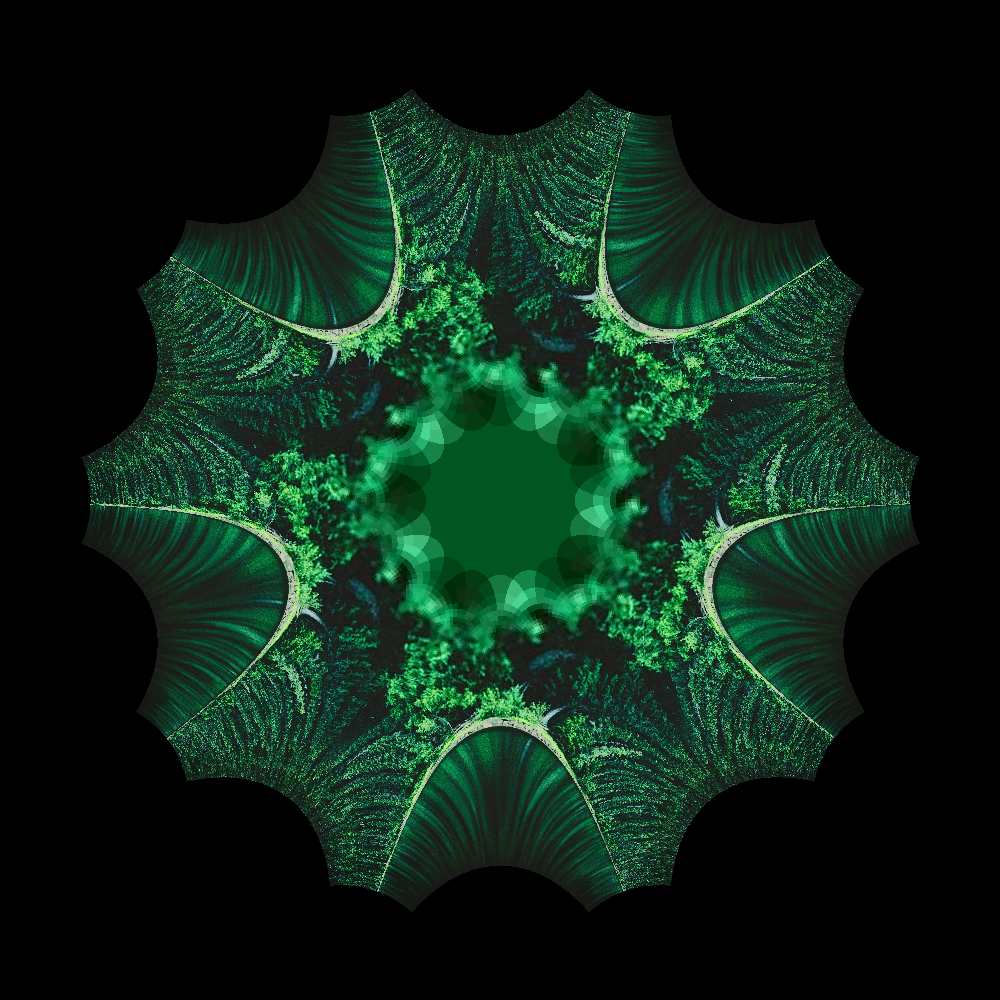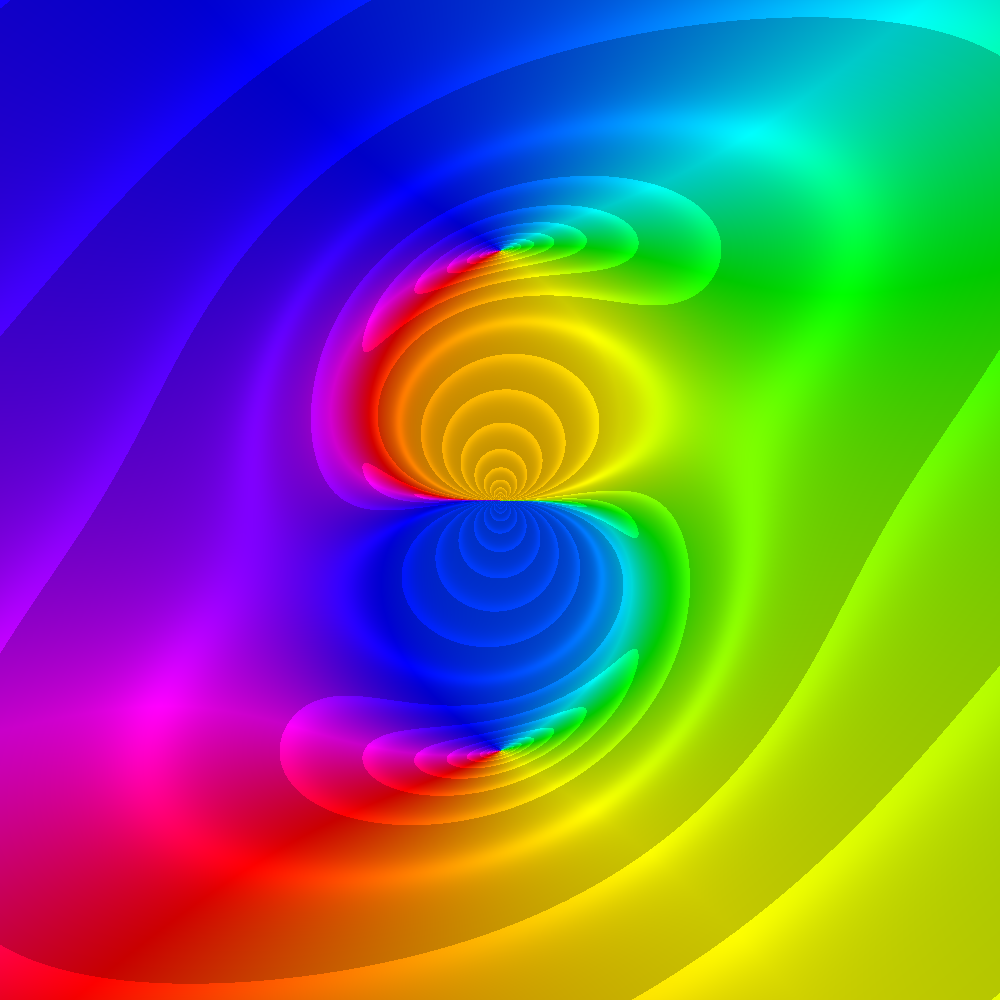Transformations from the complex plane $$\mathbb{C}$$ to itself can create beautifiul patterns and images in a simple way. First let $$S$$ be the set of all colors, and define two functions:

• a complex transformation $$f:\mathbb{C}\to\mathbb{C}$$, and
• a domain coloring scheme $$g:\mathbb{C}\to S$$.

Then, color the plane with the new coloring map $$g\circ f$$.

Different domain coloring schemes can drastically change the resulting image. Many use some combination of increments in phase, modulus, real, and imaginary coordinates. However, you can upload any image to use as a domain coloring scheme!

## Gallery# $$f(z)=z^5$$# $$f(z)=z^{4z^{3z^{2z^{z}}}}$$# $$f(z)=\frac{i}{z}-\frac{z}{i}$$